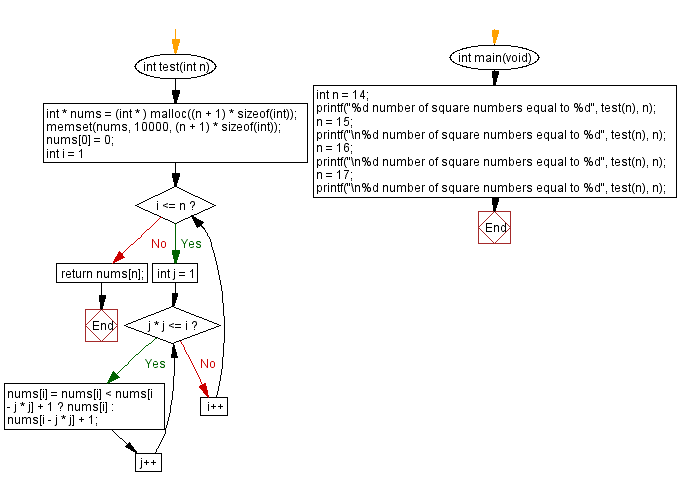﻿ C - Perfect square

# C Programming: Perfect square

## C Programming Mathematics: Exercise-29 with Solution

Write a C programming to get the smallest number of square numbers that add up to an integer n.

In mathematics, a perfect square is a number that can be expressed as either the product of an integer by itself or as the second exponent of an integer..

Sample Data:
14 = 32 + 22 + 12
Output – 3
15 = 32 + 22 + 12 + 12
Output - 4
16 = 42
Output – 1
17 = 42 + 12
Output – 2

Sample Solution:

C Code:

``````#include <stdio.h>
#include <stdlib.h>
#include <mem.h>
int test(int n) {
int * nums = (int * ) malloc((n + 1) * sizeof(int));
memset(nums, 10000, (n + 1) * sizeof(int));
nums = 0;
for (int i = 1; i <= n; i++)
for (int j = 1; j * j <= i; j++)
nums[i] = nums[i] < nums[i - j * j] + 1 ? nums[i] : nums[i - j * j] + 1;
return nums[n];
}

int main(void) {
int n = 14;
printf("%d number of square numbers equal to %d", test(n), n);
n = 15;
printf("\n%d number of square numbers equal to %d", test(n), n);
n = 16;
printf("\n%d number of square numbers equal to %d", test(n), n);
n = 17;
printf("\n%d number of square numbers equal to %d", test(n), n);
}
```
```

Sample Output:

```3 number of square numbers equal to 14
4 number of square numbers equal to 15
1 number of square numbers equal to 16
2 number of square numbers equal to 17
```

Flowchart:C Programming Code Editor:

Improve this sample solution and post your code through Disqus.

What is the difficulty level of this exercise?

Test your Programming skills with w3resource's quiz.

﻿